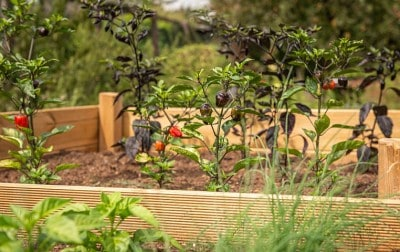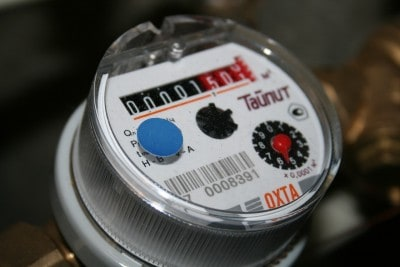# What Is Volume Used For? (10 Real Life Uses Of Volume)

Volume is used often in mathematics for word problems in geometry and calculus. However, volume also has numerous applications in real life.

So, what is volume used for?  Volume is used for many applications in business, housing, construction, gardening, physics, weather, and energy. For example, a gardener uses volume to find out how much soil to use to fill a container. An HVAC technician uses volume as one factor to determine how big a heating system needs to be.

Of course, volume has many more applications in real life: from cooking to packaging, volume plays a role.

In this article, we’ll talk about 10 important real life uses of volume.  We’ll also give some examples so you can see the numbers at work.

## What Is Volume Used For? (10 Real Life Uses Of Volume)

Volume has many applications in business, housing, construction, farming, physics, weather, energy, and other disciplines.  Here are 10 ways that volume is used in real life:

• Baking & Cooking (Volume for Measurement of Ingredients)
• Filling A Pool (Time Required)
• Gardening (Watering, Hydroponics, & Soil For Containers)
• Health & Fitness (Water Consumption)
• HVAC (Heating & Cooling)
• Medicine (Amount of a Drug)
• Physics (Density = Mass / Volume)
• Product Packaging (Dimensions of Boxes)
• Shipping & Transportation (Fuel & Transport Capacity)
• Water Bills (Price Per Gallon)

Let’s take a closer look at each of these in turn, starting with baking & cooking.

### Baking & Cooking (Volume for Measurement of Ingredients)

Volume is often used for measuring both dry ingredients (like flour, sugar, or salt) and wet ingredients (like water, milk, or oil) in baking & cooking.

For example, a recipe might call for:

• 1 cup of diced onions
• 1 cup of chopped carrots
• 1 cup of chopped celery
• 2 cups of water
• 1 tablespoon of salt

All of these ingredients are measured in volume (cups and tablespoons).  We can also figure out about how much volume this recipe will take up.

The total volume will be 3 cups (for the vegetables) plus 2 cups (for the water), or 5 cups total.  The 1 tablespoon of salt is a small volume, and some of the water will boil off as steam when cooking the soup.

So, the recipe will take up a volume of 5 cups.  To be safe, you would want a container with a volume of 6 cups or more.

### Filling A Pool (Time Required)

When filling a pool with water, you can figure out how long it will take to fill, based on the flow rate of the hose and the volume of the pool.  Volume of the pool can also tell us what kind of filtration system we need (a bigger system for a bigger pool).The time it takes to fill a swimming pool is the volume of the pool divided by the flow rate of the hose.

#### Example: Using Volume To Calculate The Time To Fill A Pool

Let’s say that you have a pool with a length of 30 feet, a width of 10 feet, and a depth of 6 feet.  Then the volume of the pool is:

• Volume = Length*Width*Depth
• Volume = 30*10*8
• Volume = 2,400 cubic feet

There are about 7.48 gallons of water in a cubic foot, so the volume of the pool in gallons is (2,400 cubic feet)*(7.48 gallons per cubic foot) = 17,952 gallons.

Let’s say that you have a garden hose with a flow rate of 10 gallons per minute.  This means that to fill the pool, it would take:

• Time = Volume / Flow Rate
• Time = 17,952 / 10
• Time = 1,795.2 minutes

If we divide this result by 60, we get 1795.2 / 60 = 29.92 hours.  So, it will take about 1.25 days to fill up the pool completely.

### Gardening (Watering, Hydroponics, & Soil For Containers)

Volume is used for several purposes in gardening:

• Watering: if we know how much water a plant needs and the flow rate of the hose, we can figure out how long to water the plant (volume / flow rate)
• Hydroponics/Aquaponics: in a soilless growing system, we will need a filter that can handle the volume of water that we want to filter.
• Soil for Containers: when you fill up a pot or raised bed, you need to know the volume to figure out how much soil or potting mix you will need.A raised bed will require a volume of soil to fill, depending on the dimensions of the bed.

#### Example: Calculating Soil Needed For A Raised Garden Bed

Let’s say you have a raised garden bed with a length of 10 feet, a width of 4 feet, and a depth of 3 feet.  Let’s also assume that you only want to fill the raised bed to a depth of 2 feet.

Then the volume of soil you would need is:

• Volume = Length*Width*Depth
• Volume = 10*4*2
• Volume = 80 cubic feet

So, you would need 80 cubic feet of soil to fill this raised garden bed.  A cubic yard is 27 cubic feet (3*3*3 = 27), so you would need about 3 cubic feet of soil (81 / 27 is about 3).

### Health & Fitness (Water Consumption)

If you are trying to stay healthy, drinking plenty of water is a good idea.  It will keep you hydrated during workouts, and it is better than drinking sugary soft drinks.

The Mayo Clinic recommends drinking 0.5 to 1 ounce of water daily for every pound that you weigh.  So, if you weigh 150 pounds, you should drink 75 to 150 ounces of water per day.

If you exercise a lot and live in a hot, dry climate, you might want to be on the high side (perhaps 150 ounces per day).  If you drink water 5 times per day (breakfast, mid-morning, lunch, afternoon, dinner), then you will need to drink 150 / 5 = 30 ounces of water each time (about ¼ gallon, or 4 cups).

### HVAC (Heating & Cooling)

In order to heat or cool the air in a space, it takes a certain amount of energy.  The larger the volume in the space, the more powerful the heating or cooling system needs to be.

The volume of a space will give us an idea of how big the system should be.

#### Example: Finding The Volume Of A House

Let’s say that your house has an area of 1,500 square feet, with ceilings that are 8 feet high.  Then the volume of the house is:

• Volume = Area*Height
• Volume = 1,500*8
• Volume = 12,000 cubic feet

Generally, an area this size will need a heating system with 80,000 BTU (depending on your home’s insulation, how much sun exposure your house gets, and how cold your climate gets in the winter). Your HVAC technician can make specific recommendations for your situation.

### Medicine (Amount of a Drug)

In medicine, volume has many uses, including:

• calculating how much of a drug to give a patient (this may depend on weight)
• calculating how much blood a patient needs for a transfusion (depending on how much was lost)
• calculating how much air is needed for artificial ventilation (an adult would need more air than a child)

### Physics (Density = Mass / Volume)

In physics, volume is used to calculate the density of objects (you can learn more about what factors affect density in my article here).  The density of an object is given by:

• Density = Mass / Volume

where mass is generally in kilograms and volume is in cubic meters (so density is in kilograms per cubic meter).  However, we might also see units of grams per cubic centimeter.

#### Example: Density Of Two Materials

Let’s say that we have a slab of lead that is 2 centimeters by 5 centimeters by 10 centimeters, with a total volume of 2*5*10 = 100 cubic centimeters.  The slab has a mass of 1129 grams, which means that the density is:

• Density = Mass / Volume
• Density = 1129 grams / 100 cubic centimeters
• Density = 11.29 grams per cubic centimeter

Let’s say that we also have a container full of liquid mercury (near room temperature).  There are 200 cubic centimeters of mercury in the container, and the mass is 2707 grams.

Then the density of the mercury is:

• Density = Mass / Volume
• Density = 2707 grams / 200 cubic centimeters
• Density = 13.535 grams per cubic centimeter

This tells us that mercury is more dense than lead.  In fact, the slab of lead will float in the liquid mercury, since the lead has a lower density!

### Product Packaging (Dimensions of Boxes)

We can use volume to optimize that size of a box used for packaging products.  This will cut down on waste and save space when shipping these boxes.

You can also figure out the best way to pack several boxes into a truck to optimize transportation costs.

### Shipping & Transportation (Fuel & Transport Capacity)

When shipping by air, sea, or land, you will need to pack the truck or container as full as you can (use up the volume) without overloading it (maximum weight and weight distribution).The fuel needed for a trip depends on the fuel efficiency (gas mileage) and distance to travel.

You will also need fuel to get things where they need to go.  If you divide the distance to travel by the mileage (fuel efficiency) of the vehicle, you will get the volume of fuel needed.

#### Example: Volume Of Fuel For Transportation

Let’s say that your truck gets 15 miles per gallon.  If you have to travel a distance of 450 miles, then you will need:

• Volume of Fuel = Distance / Vehicle Gas Mileage
• Volume of Fuel = 450 miles / 15 miles per gallon
• Volume of Fuel = 30 gallons

So, you would need about 30 gallons of fuel for the trip (plus another 30 gallons for the return trip, and perhaps a little extra for unforeseen emergencies, such as detours).

### Water Bills (Price Per Gallon)

Your water bill is usually determined by two factors:

• A minimum price (perhaps \$10 per month)
• A charge for volume of water used (sometimes the rate increases at various amounts)

#### Example: Water Bill Calculation Based On Volume

Let’s say that your water bill has a minimum charge of \$10 per month.  You would add the following charges for volume:

• 0 to 100 cubic feet: \$0.01 per cubic foot
• 101 to 500 cubic feet: \$0.02 per cubic foot
• 501 to 1000 cubic feet: \$0.05 per cubic foot
• Over 1000 cubic feet: \$0.10 per cubic foot

So, if you use 400 cubic feet of water this month, your bill would be:

• \$10 (base charge) + 100(\$0.01) (first 100 cubic feet) + 300(\$0.02) (next 300 cubic feet)
• =\$10 + \$1 + \$6
• =\$17A water meter records the volume of water that you use in a given time period.

## Conclusion

Now you know some of the uses of volume in real life, along with how to do some of the calculations for those applications.

You can learn about various uses of algebra in real life here.